Courses

# Test: Power Systems- 2

## 20 Questions MCQ Test GATE Electrical Engineering (EE) 2022 Mock Test Series | Test: Power Systems- 2

Description
This mock test of Test: Power Systems- 2 for GATE helps you for every GATE entrance exam. This contains 20 Multiple Choice Questions for GATE Test: Power Systems- 2 (mcq) to study with solutions a complete question bank. The solved questions answers in this Test: Power Systems- 2 quiz give you a good mix of easy questions and tough questions. GATE students definitely take this Test: Power Systems- 2 exercise for a better result in the exam. You can find other Test: Power Systems- 2 extra questions, long questions & short questions for GATE on EduRev as well by searching above.
QUESTION: 1

### Three generators rated 100 MVA, 11 kV have an impedance of 0.15pu each. If in the same plant, these generators are being replaced by a single equivalent generator, the effective impedance of equivalent generator will be

Solution:

Applying Thevenin's theorm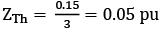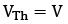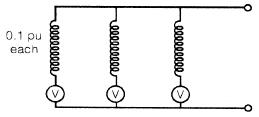QUESTION: 2

### If all the sequence voltages at the fault point in a power system are equal, then the fault is a

Solution:

For a solid fault i.e, Zf = 0, Va1 = Va2 = Va0 for L-L-G fault.

QUESTION: 3

### A three-phase transformer having zero-sequence impedance of Z0 has the zero-sequence network as shown in the figure below. The connections of its windings are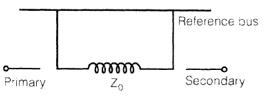Solution:
QUESTION: 4

Which one of the following relays has the capability of anticipating the possible major fault in a transformer?

Solution:

Buchholz relay takes care of all incipient internal faults.

QUESTION: 5

In a 220 kV system, the inductance and capacitance up to the circuit breaker location are 25 mH and 0.025 μF respectively. The value of resistor required to be connected across the breaker contacts which will give no transient oscillations, is

Solution: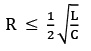or no transient oscillation.

QUESTION: 6

The operating characteristic of a distance relay in the R-X plane is shown in the figure below, It represents operating characteristic of a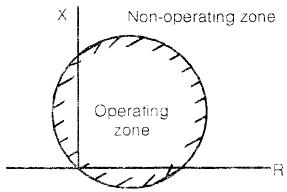Solution:
QUESTION: 7

Two power plants interconnected by a tie-line as shown in the below figure have loss formula coefficient B11 = 10-3 MW-1. Power is being dispatched economically with plant '1' as 100 MW and plant ꞌ2' as 125 MW. The penalty factors for plants 1 and 2 are respectively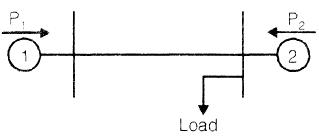Solution:

Since the load is at the bus of plant-2, therefore, the line loss will not be affected by variation of P2 . Thus B12 = B21 = 0 and B22 = 0
For a 2-plant system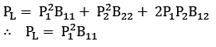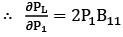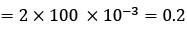∴ Penalty factor for plant-1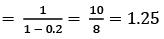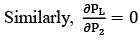∴ Penalty factor for plant-2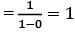Penalty factor is given by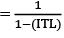Where ITL means incremental transmission loss.

QUESTION: 8

The below diagram shows the layout of a power station having two generators A and B, connected to the 11 kV buses which are also fed through two transformers C and D from a 132 kV grid. The 11 kV buses are interconnected through a reactor R.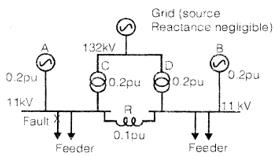The reactance of A, B, C, D and R in p.u. on a common MVA and kV-base. All the generated voltages in A, B and grid are each 1.0 p.u. and assumed as in phase at the time of fault.
The steady state symmetrical fault-current for a 3-phase fault on the 11 kV feeders is

Solution: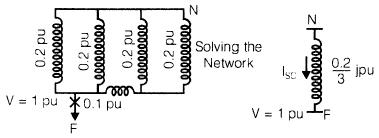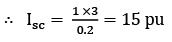QUESTION: 9

The speed regulation parameter R of a control area is 0.025 Hz/MW and load frequency constant D is 2 MW/Hz, The area frequency response characteristic (AFRC) is

Solution: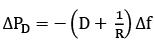∴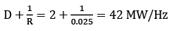Where, Δf → change in frequency and ΔPD → change in load demand.

QUESTION: 10

The per-unit impedance of a circuit element of 0.15. If the base kV and base MVA are halved, then the new value of the per-unit impedance of the circuit element will be

Solution: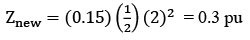QUESTION: 11

The per-unit impedance of an alternator corresponding to base values 13.2 kV and 30 MVA is 0.2 p.u. The p.u. value of the impedance for base values 13.8kV and 50 MVA in p.u. will be

Solution: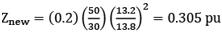QUESTION: 12

For a given base voltage and base volt-amp, the per unit impedance value of an element is X. What will be the per-unit impedance value of this element when the voltage andvolt-amp bases are both doubled?

Solution: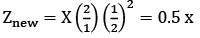QUESTION: 13

The reflection coefficient for the transmission line shown in figure below at point P is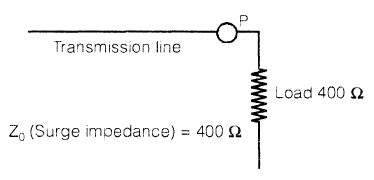Solution:

The line is terminated into its surge impedance so it is an infinite line.

QUESTION: 14

A 3-phase transmission line has its conductors at the corners of an equilateral triangle with side 3 m. The diameter of each conductor is 1.63 cm. The inductance of the line per phase per km is

Solution: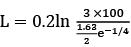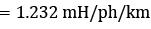QUESTION: 15

A cable has inductance of 0.22 mH per km and capacitance of 0.202 μF per km. The surge impedance of the cable is

Solution:

Z0 =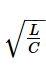QUESTION: 16

For some given transmission line the expression for voltage regulation is given by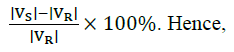Solution:

In case of a short line, when load is removed, the voltage at the receiving end is equal to the voltage at the sending end. At full load |VR, Fl| = |VR| and at no load, |VR, nl| = |VS|

QUESTION: 17

In a certain single-phase a.c. circuit the instantaneous voltage is given by v = V sin (ωt + 30°) p.u. and the instantaneous current is given by i = I sin (ωt - 30°) p.u. Hence the per unit value of reactive power is

Solution: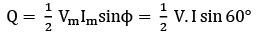QUESTION: 18

A surge of l00 kV travels along an overhead line towards its junction with a cable. The surge impedance for the overhead line and cable are 400 ohms and 50 ohms respectively. The magnitude of the surge transmitted through the cable is

Solution: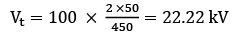QUESTION: 19

A surge voltage rising at 100 kV/μs travels along a loss-less open-circuited transmission line. It takes 10 μs to reach the open end. The reflected wave from the open end, will be rising at

Solution:

VR = Vi =  100 × 10 = 1000V/µs

As Z2 = ∞

QUESTION: 20

At slack bus, which one of the following combinations of variables is not specified?

Solution:

Load bus: Known quantities - P and Q Unknown quantities - V and δ

Generator bus: Known quantities - P and V Unknown quantities - Q and δ

Slack or reference bus: Known quantities - V and δ Unknown quantities - P and Q